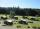# Roses

On the large rosary was a third white, half red, yellow quarter and six pink. How many roses was in the rosary?

Result

x =  -72

#### Solution:

x/3+x/2+x/4+6=x

x = -72

x = -72

Calculated by our simple equation calculator.

Leave us a comment of example and its solution (i.e. if it is still somewhat unclear...):

Showing 0 comments:Be the first to comment!#### To solve this example are needed these knowledge from mathematics:

Need help calculate sum, simplify or multiply fractions? Try our fraction calculator. Do you have a linear equation or system of equations and looking for its solution? Or do you have quadratic equation?

## Next similar examples:

1. Three monksThree medieval monks has task to copy 600 pages of the Bible. One rewrites in three days 1 page, second in 2 days 3 pages and a third in 4 days 2 sides. Calculate for how many days and what day the monks will have copied whole Bible when they begin Wednesd
2. Sales storesThe first sales store passed the 1/3 and the second 2/5 of the total amount of goods.In third store passed the 2/3 rest of the goods. The remaining 40 kg of goods put into fourth store. How many kilograms of goods pass to a third store?
3. Unknown numberI think the number - its sixth is 3 smaller than its third.
4. Cleaning windowsCleaning company has to wash all the windows of the school. The first day washes one-sixth of the windows of the school, the next day three more windows than the first day and the remaining 18 windows washes on the third day. Calculate how many windows ha
5. UN 1If we add to an unknown number his quarter, we get 210. Identify unknown number.
6. ResortIn a completely crowded resort was 30% from the Czech Republic quarter visitors were from Slovakia and the rest 135 came from Germany. What capacity have resort?
7. FractionsThree-quarters of an unknown number are 4/5. What is 5/6 of this unknown number?
8. PearsThere were pears in the basket, I took two-fifths of them, and left six in the basket. How many pears did I take?
9. EquationSolve the equation: 1/2-2/8 = 1/10; Write the result as a decimal number.
10. Simple equationSolve for x: 3(x + 2) = x - 18
11. Fifth of the numberThe fifth of the number is by 24 less than that number. What is the number?
12. Simple equation 6Solve equation with one variable: X/2+X/3+X/4=X+4
13. Unknown numberIdentify unknown number which 1/5 is 40 greater than one tenth of that number.
14. CandyPeter had a sachet of candy. He wanted to share with his friends. If he gave them 30 candies, he would have 62 candies. If he gave them 40 candies, he would miss 8 candies. How many friends did Peter have?
15. Equation with xSolve the following equation: 2x- (8x + 1) - (x + 2) / 5 = 9
16. EqnSolve equation with fractions: 2x/3-50=40+x/4
17. Equation with fractionsSolve equation: ? It is equation with fractions.